## 请教个c++模板类编译问题

coolge 发布于 2012/07/08 09:58

```template<typename T,typename U>
class ReferenceCount
{
public:
ReferenceCount(){}
~ReferenceCount(){}
void func(T& a,U b);
}

template <typename requestT,typename responseT>
//ReferenceCount 也是一个模板类
class A:public ReferenceCount<A<requestT,responseT>,int>
{
public:
A(){}
A(requestT a,responsetT b)();
virtual ~A(){}
}

int main()
{
typea a;
typeb b;
A<typea,typeb>(a,b);
....
return 0;
}```

0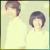```template<typename T,typename U>
class ReferenceCount
{
public:
ReferenceCount(){}
~ReferenceCount(){}
void func(T& a,U b);
};
template<typename T,typename U>
void ReferenceCount<T , U>::func(T& a,U b)
{

}
template <typename requestT,typename responseT>
//ReferenceCount 也是一个模板类
class A:public ReferenceCount<A<requestT,responseT>,int>
{
public:
A(){}
//A(requestT a,responseT b)();
virtual ~A(){}
};

int main()
{
// 	int  a;
// 	typeb b;
A<int,int>();

return 0;
}```

0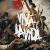1. 换高级点的gcc看看. 4.1还是很偏老了. gcc 3系列对付C++很差, 这4.1离3也不远. =.=||

2. `class` `A:``public` `ReferenceCount<A<requestT,responseT>,``int``> => ``class` `A:``public` `ReferenceCount<typename A<requestT,responseT>,``int``> 看能不能帮gcc4.1理解理解. `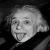00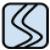ReferenceCount作为基类，A类又是public继承，所以ReferenceCount惜购函数必须是virtual否则无法正确 析构子类，行为不确定。

```template<typename T,typename U>
class ReferenceCount
{
public:
ReferenceCount(){}
virtual ~ReferenceCount(){}
void func(T& a,U b);
}```

0

```template<typename T,typename U>
class ReferenceCount
{
public:
ReferenceCount(){}
~ReferenceCount(){}
void func(T& a,U b);
};

template <typename requestT,typename responseT>
//ReferenceCount 也是一个模板类
class A:public ReferenceCount<A<requestT,responseT>,int>
{
public:
A(){}
A(requestT a,responsetT b)();
virtual ~A(){}
};

class typea { };
class typeb { };

int main()
{
typea a;
typeb b;
A<typea,typeb> c(a,b);
return 0;
}```

VS2010产生如下错误

0

template<typename T,typename U>
class ReferenceCount
{
public:
ReferenceCount(){}
~ReferenceCount(){}
void func(T& a,U b);
};

template <typename requestT,typename responseT>
//ReferenceCount 也是一个模板类
class A:public ReferenceCount<A<requestT,responseT>,int>
{
public:
A(){}
A(requestT a,responsetT b)();
virtual ~A(){}
};

class typea { };
class typeb { };

int main()
{
typea a;
typeb b;
A<typea,typeb> c(a,b);
return 0;
}

VS2010产生如下错误

```template<typename T,typename U>
class ReferenceCount
{
public:
ReferenceCount(){}
~ReferenceCount(){}
void func(T& a,U b);
};

template <typename requestT,typename responseT>
//ReferenceCount 也是一个模板类
class A:public ReferenceCount<A<requestT,responseT>,int>
{
public:
A(){}
A(requestT a,responsetT b)();
virtual ~A(){}
};

class typea { };
class typeb { };

int main()
{
typea a;
typeb b;
A<typea,typeb> c(a,b);
return 0;
}```

VS2010产生如下错误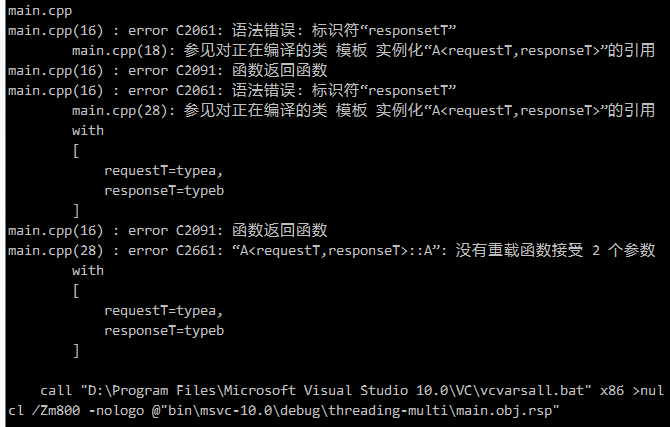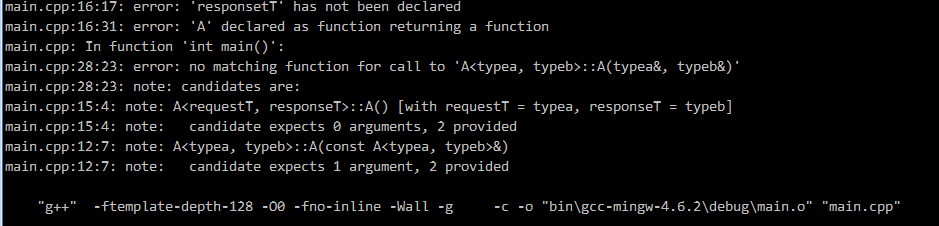0
```template<typename T,typename U>
class ReferenceCount
{
public:
ReferenceCount(){}
~ReferenceCount(){}
void func(T& a,U b);
};

template <typename requestT,typename responseT>
//ReferenceCount 也是一个模板类
class A:public ReferenceCount<A<requestT,responseT>,int>
{
public:
A(){}
A(requestT & a,responsetT & b) { }
virtual ~A(){}
};

class typea { };
class typeb { };

int main()
{
typea a;
typeb b;
A<typea,typeb> c(a,b);
return 0;
}```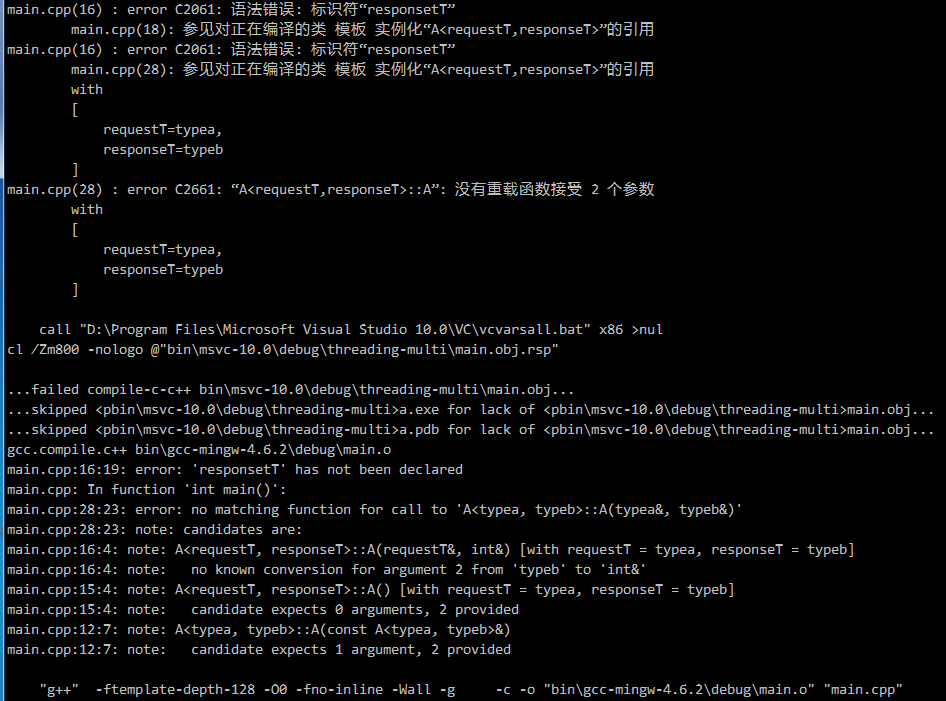A(requestT & a,responsetT & b) { } to A(requestT & a,responseT & b) { }
0
```template<typename T,typename U>
class ReferenceCount
{
public:
ReferenceCount(){}
~ReferenceCount(){}
void func(T& a,U b);
};

template <typename requestT,typename responseT>
//ReferenceCount 也是一个模板类
class A:public ReferenceCount<A<requestT,responseT>,int>
{
public:
A(){}
A(requestT & a,responseT & b) { }
virtual ~A(){}
};

class typea { };
class typeb { };

int main()
{
typea a;
typeb b;
A<typea,typeb> c(a,b);
return 0;
}```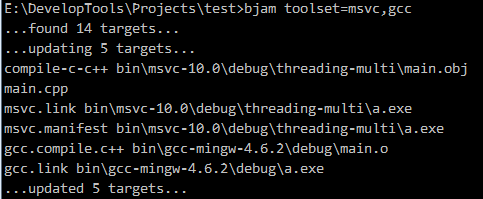0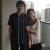```template<typename T,typename U>
class ReferenceCount
{
public:
ReferenceCount(){}
~ReferenceCount(){}
void func(T& a,U b);
};

template <typename requestT,typename responseT>
//ReferenceCount 也是一个模板类
class A:public ReferenceCount<A<requestT,responseT>,int>
{
public:
A(){}
A(requestT a,responseT b){};
virtual ~A(){}
};

int main()
{
int a;
double b;
A<int,double>(a,b);

return 0;
}```